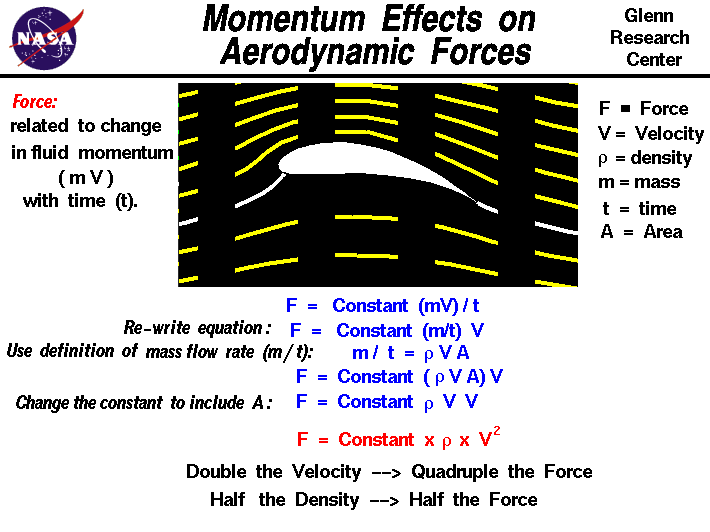+ Text Only Site
+ Non-Flash Version
+ Contact GlennLift is created by deflecting a flow of air, and drag is generated on a body in a wide variety of ways. From Newton's second law of motion, the aerodynamic force F on the body is directly related to the change in momentum of the fluid with time t. The fluid momentum is equal to the mass m times the velocity V of the fluid. F = d (m * V) / dt F = constant * V * m / t Since the air moves, defining the mass is tricky. If the mass of fluid were brought to a halt, it would occupy some volume in space. We can define the density (r) of the fluid to be the mass divided by the volume v. r = m / v Since the fluid is moving, we must determine the mass in terms of the mass flow rate. The mass flow rate is the amount of mass passing a given point during some time interval t and its units are mass/time. We can relate the mass flow rate to the density mathematically. The mass flow rate mdot is equal to the density times the velocity times the area A through which the mass passes. mdot = m / t = r * V * A With knowledge of the mass flow rate, we can express the aerodynamic force as equal to the mass flow rate times the velocity. F = constant * V * r * V * A A quick units check: mass * length / time^2 = constant * length/time * mass/length^3 * length/time * length^2 mass * length / time^2 = mass * length/time^2 Combining the velocity dependence and absorbing the area into the constant, we find: F = constant * r * V^2 The aerodynamic force equals a constant times the density times the velocity squared. The dynamic pressure of a moving flow is equal to one half of the density times the velocity squared. The aerodynamic force is directly proportional to the dynamic pressure of the flow. Effect of Velocity on Aerodynamic Forces The velocity used in the aerodynamic equation is the relative velocity between an object and the flow. The aerodynamic force depends on the square of the velocity. Doubling the velocity quadruples the force. The dependence of lift and drag on the square of the velocity has been known for more than a hundred years. The Wright brothers used this information in the design of their first aircraft. Effect of Air Density on Aerodynamic Forces The aerodynamic force depends linearly on the density of the air. Halving the density halves the force. As altitude increases, the air density decreases. This explains why airplanes have a flight ceiling, an altitude above which it cannot fly. As an airplane ascends, a point is reached where there is not enough air mass to generate enough lift to overcome the airplane's weight. The relation between altitude and density is a fairly complex exponential. FoilSim Applet You can investigate the effect of momentum on lift by using the FoilSim II Java Applet. Set a small angle of attack using the slider, then vary the "Speed" and "Altitude." Try doubling the speed and notice the effect on lift. Change the altitude until the air density is half of its previous value. What happened to the lift? You can use the browser "Back" button to return to this page. If your browser does not support Java, or you just want your own copy of FoilSim to play with, you can download it at no charge. Activities:Guided ToursLift Equation:Drag Equation:Navigation ..Beginner's Guide Home Page+ Inspector General Hotline + Equal Employment Opportunity Data Posted Pursuant to the No Fear Act + Budgets, Strategic Plans and Accountability Reports + Freedom of Information Act + The President's Management Agenda + NASA Privacy Statement, Disclaimer, and Accessibility CertificationEditor: Nancy Hall NASA Official: Nancy Hall Last Updated: May 05 2015 + Contact Glenn